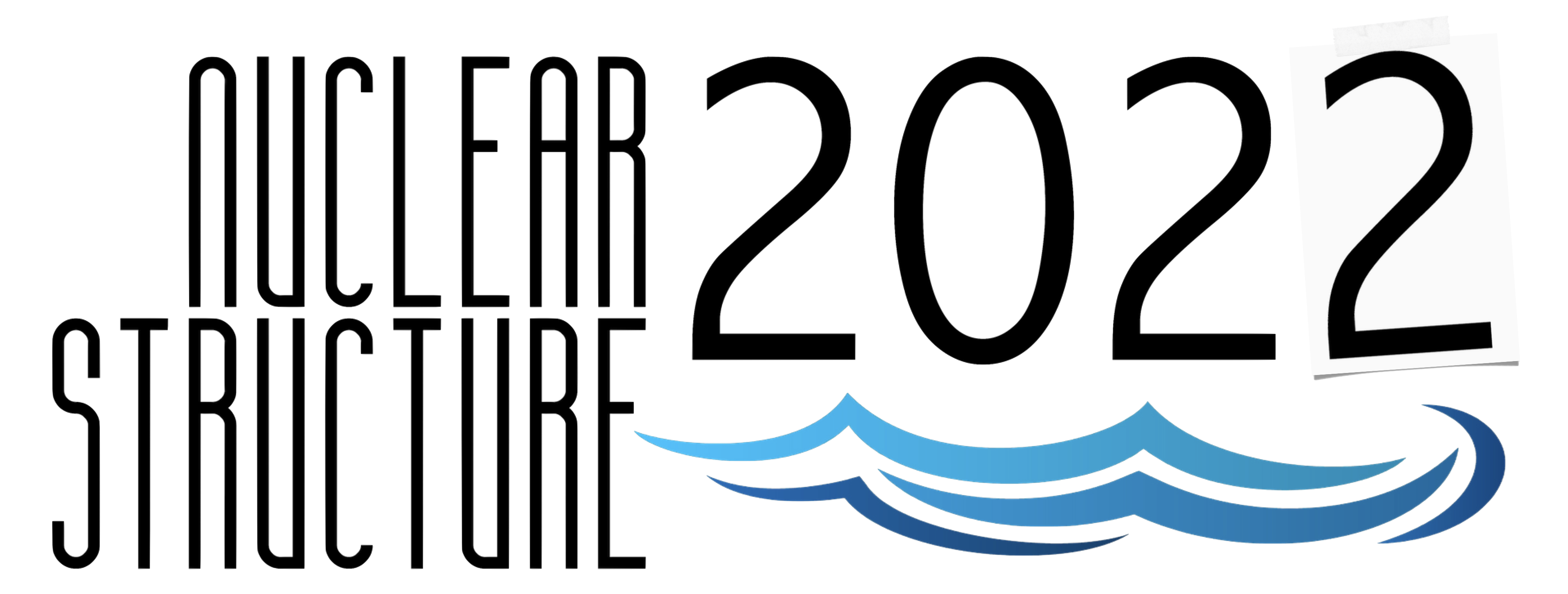#Nuclear Structure 2022

Jun 13 – 17, 2022
Berkeley, CA
US/Pacific timezone

## Spectroscopy of Trans-fermium Nuclei Using the Argonne Gas-Filled Analyzer

Jun 13, 2022, 11:30 AM
20m
Berkeley, CA

#### Berkeley, CA

Lawrence Berkeley National Laboratory
Oral Oral Presentations

### Speaker

D. Seweryniak (Physics Division, Argonne National Laboratory, Argonne, IL 60439)

### Description

Spectroscopy of Trans-fermium Nuclei Using the Argonne Gas-Filled Analyzer

D. Seweryniak,$^1$ T. Huang,$^{1,2}$ K. Auranen,$^{1,11}$ A.D. Ayangeakaa,$^{1,12}$ B.B. Back,$^1$ P. Bender,$^3$
M.P. Carpenter,$^1$ P. Chowdhury,$^3$ R.M. Clark,$^4$ P. Copp,$^1$ Z. Favier,$^{10}$ K. Hauschild,$^8$ X.-T. He,$^5$
R.D. Herzberg,$^6$ D. Ho,$^3$ H. Jayatissa,$^1$ T.L. Khoo,$^1$ F.G. Kondev,$^1$ G. Morgan,$^7$ C. Morse,$^4$
A. Korichi,$^8$ T. Lauritsen,$^1$ J. Li,$^1$ C. Mueller-Gatermann,$^1$ D. Potterveld,$^1$ W. Reviol,$^1$ A. Rogers,$^3$ S. Saha,$^3$ G. Savard,$^1$ K. Sharma,$^3$ S. Stolze,$^1$ S. Waniganeththi,$^3$ G. Wilson,$^1$ J. Wu,$^1$ Y.-F. Xu,$^5$,
S. Zhu$^9$

$^1$Argonne National Laboratory, Argonne, IL 60439, USA
$^2$Institute of Modern Physics, Chinese Academy of Sciences, Lanzhou 730000, China
$^3$University of Massachusetts, Lowell, MA 01854, USA
$^4$Lawrence Berkeley National Laboratory, Berkeley, CA 94720, USA
$^5$College of Materials Science and Technology, Nanjing University of Aeronautics and Astronautics, Nanjing 210016, China
$^6$University of Liverpool, Liverpool, UK
$^7$Louisiana State University, Baton Rouge, LA 70803, USA
$^8$CSNSM, IN2P3-CNRS Orsay, France
$^9$Brookhaven National Laboratory, Upton, NY 11973, USA
$^{10}$CEA, Département de Physique Nucléaire, Université Paris-Saclay, 91191, Gif-sur-Yvette, France
$^{11}$Accelerator Laboratory, Department of Physics, University of Jyväskylä, FI-40014 Jyväskylä, Finland
$^{12}$Triangle Universities Nuclear Laboratory, Duke University, Durham, North Carolina 27708, USA

Deformed nuclei near the Z=100, N=152 deformed shell gaps are a stringent testing ground for nuclear models which are used to describe the heaviest known nuclei. Nuclei in this region have been studied using in-beam, K-isomer, $\alpha$-decay and spontaneous fission spectroscopic methods. To extend these studies to odd-A, odd-odd, and to heavier nuclei the Argonne Gas-Filled Analyzer (AGFA) was constructed. During the talk, recent decay and isomer spectroscopy experiments with AGFA in stand-alone mode and in-beam spectroscopy experiments with AGFA coupled to the Gammasphere $\gamma$-ray detector array will be reviewed. Among others, the observation of the ground-state rotational band in the fissile nucleus $^{254}$Rf and the discovery of the new isotope $^{251}$Lr will be discussed. The moment of inertia deduced for $^{254}$Rf shades light on the shape evolution in this region while the observed $\alpha$-decay fine structure in $^{251}$Lr provides information on single-proton orbitals near the Fermi surface. Plans for experimental program with AGFA will be also presented.

This material is based upon work supported by the U.S Department of Energy, Office of Science, Office of Nuclear Physics, under contract number DE-AC02-06CH11357. This research used resources of ANL's ATLAS facility, which is a DOE Office of Science User Facility.

### Primary author

D. Seweryniak (Physics Division, Argonne National Laboratory, Argonne, IL 60439)

### Presentation materials

There are no materials yet.